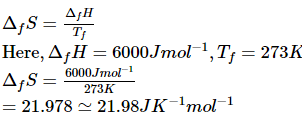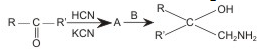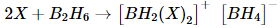# WBJEE Chemistry Test - 3

## 40 Questions MCQ Test WBJEE Sample Papers, Section Wise & Full Mock Tests | WBJEE Chemistry Test - 3

Description
Attempt WBJEE Chemistry Test - 3 | 40 questions in 60 minutes | Mock test for JEE preparation | Free important questions MCQ to study WBJEE Sample Papers, Section Wise & Full Mock Tests for JEE Exam | Download free PDF with solutions
QUESTION: 1

Solution:
QUESTION: 2

Solution:
QUESTION: 3

### Schiff's reagent gives pink colour with

Solution:
QUESTION: 4
Which of the nitrates on strong heating leaves the metal as the residue?
Solution:
QUESTION: 5
Which of the following does not show electromeric effect?
Solution:
QUESTION: 6

Which one of the following molecules has the smallest bond angle?

Solution:

Molecules      Bond angle
NH3        -       107.2º
PH3        -       93.2º
H2O        -       104.45º
H2Se       -       90.6º
H2S        -       92.1º

QUESTION: 7
Concentrated nitric acid is added before proceeding to test for group III members. This is to
Solution:
QUESTION: 8
What is the maximum number of electrons that can be accommodated in an atom in which the highest principal quantum number value is 4?
Solution:
QUESTION: 9
In DNA the complementary beses are
Solution:
QUESTION: 10

The heat of formation of the compound in the following reaction is
H₂(g) + Cl(g)→ 2HCl(g)+44 Kcal

Solution:
QUESTION: 11
The equilibrium between water and its vapour, in an open-vessel
Solution: In an open vessel, the water vapour diffuse into air and therefore, the concentration of water vapour above water surface is negligible.
The reverse process of condensation of vapour into liquid form is almost absent.
Therefore, evaporation process continues, till the entire water changes into vapour form.
Therefore, in an open vessel, the water vapour system is never in equilibrium.
Finally the whole water will diffuse into air as water vapour.
QUESTION: 12
Which of the following is not chiral ?
Solution:
QUESTION: 13
In a reaction 2A + B → A₂B, the reactant A will disappear at
Solution:
QUESTION: 14
Parathion is
Solution:
QUESTION: 15

What is the entropy change (in J K-1 mol-1), when one mole of ice is converted into water at 0ºC? (The enthalpy change for the conversion of ice to liquid water is 6.0kJ mol-1 at 0ºC)

Solution:QUESTION: 16
A metal M readily forms water soluble sulphate MSO₄. Water becomes inert on heating. The hydroxide is soluble in NaOH. Then M is
Solution:
QUESTION: 17
The most stable complex among the following is
Solution:
QUESTION: 18
Which of the following statement is correct with respect to the property of elements with increase in atomic number in the carbon family(group 14)?
Solution:
QUESTION: 19
What is formed when boron is heated with concentrated H₂SO₄?
Solution:
QUESTION: 20
Air at sea level is dense. This is a practical application of
Solution:
QUESTION: 21
Among the following hydrides which is more stable
Solution:
QUESTION: 22
Which one of the following metals reacts with water at room temperature ?
Solution:
QUESTION: 23
The number of optical iosmers of the compound CH₃CHBrCHBrCOOH is
Solution:
QUESTION: 24
The radiation which has highest penetrating power is
Solution:
QUESTION: 25

Aniline reacts with phosgene and KOH to form

Solution:
QUESTION: 26
The product of addition polymerization reaction is
Solution:
QUESTION: 27
Which of the following is used for making horses, shoe heels and stoppers?
Solution:
QUESTION: 28
The oxidation number of Mn in KMnO₄ is
Solution:
QUESTION: 29
In crystal structure of sodium chloride, the arrangement of Cl⁻ ions is
Solution:
QUESTION: 30
What is the number of asymmetric carbon atoms present in α-D-glucopyranose molecule ?
Solution:
QUESTION: 31

α-D-(+)-glucose and β-D-(+)-glucose are

Solution:
QUESTION: 32

The major product obtained on interaction of phenol with sodium hydroxide and carbon dioxide is

Solution:
QUESTION: 33

Among the following substituted silanes the one which will give rise to cross linked silicone polymer on hydrolysis is

Solution:
QUESTION: 34

The IUPAC name of [Ni(NH3)4] [NiCl4] is

Solution:
QUESTION: 35

A and B in the following reactions are:Solution:
*Multiple options can be correct
QUESTION: 36

The statements that are true for the long form of the periodic table are :

Solution:
*Multiple options can be correct
QUESTION: 37

For the reaction A + 3 B → C , the differential form of the rate law is

Solution:
*Multiple options can be correct
QUESTION: 38

In the reactionthe amine(s) X is(are)

Solution:
*Multiple options can be correct
QUESTION: 39

Which of the following statements is/are incorrect?

Solution:
QUESTION: 40Use Code STAYHOME200 and get INR 200 additional OFF Use Coupon Code